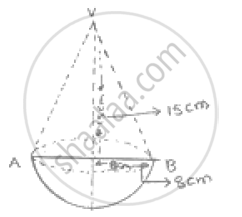Share

Books Shortlist

# A Wooden Toy is in the Form of a Cone Surmounted on a Hemisphere. the Diameter of the Base of the Cone is 16 Cm and Its Height is 15 Cm. Find the Cost of Painting the Toy at Rs. 7 per 100 Cm^2. - CBSE Class 9 - Mathematics

ConceptSurface Area of a Sphere

#### Question

A wooden toy is in the form of a cone surmounted on a hemisphere. The diameter of the base
of the cone is 16 cm and its height is 15 cm. Find the cost of painting the toy at Rs. 7 per 100
cm^2.

#### Solution 1

Diameter of cone = 16cm.
∴ Radius of cone = 8cm.
Height of cone = 15cm
Slant height of cone - sqrt(8^2+15^2)-sqrt(64+225)

-sqrt(289)

-17 cm

∴ Total curved surface area of toy

-πrl + 2πr^2

-22/7 × 8 × 17 + 2 × 22/7 × 8^2

- 5808/7cm^2

Now .cost of 100cm^2 - Rs.7

1cm^2 - Rs7/100

Hence , cost of 5808/7 cm^2 - Rs (5808/7×7/100)

-Rs.58.08.

#### Solution 2

Diameter of cone = 16cm.
∴ Radius of cone = 8cm.
Height of cone = 15cm
Slant height of cone - sqrt(8^2+15^2)-sqrt(64+225)

-sqrt(289)

-17 cm

∴ Total curved surface area of toy

-πrl + 2πr^2

-22/7 × 8 × 17 + 2 × 22/7 × 8^2

- 5808/7cm^2

Now .cost of 100cm^2 - Rs.7

1cm^2 - Rs7/100

Hence , cost of 5808/7 cm^2 - Rs (5808/7×7/100)

-Rs.58.08.

Is there an error in this question or solution?

#### APPEARS IN

RD Sharma Solution for Mathematics for Class 9 by R D Sharma (2018-19 Session) (2018 to Current)
Chapter 21: Surface Areas and Volume of a Sphere
Ex.21.10 | Q: 11 | Page no. 8
Solution A Wooden Toy is in the Form of a Cone Surmounted on a Hemisphere. the Diameter of the Base of the Cone is 16 Cm and Its Height is 15 Cm. Find the Cost of Painting the Toy at Rs. 7 per 100 Cm^2. Concept: Surface Area of a Sphere.
S